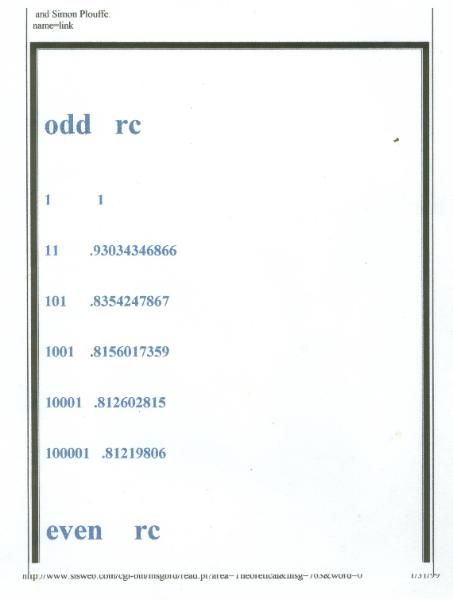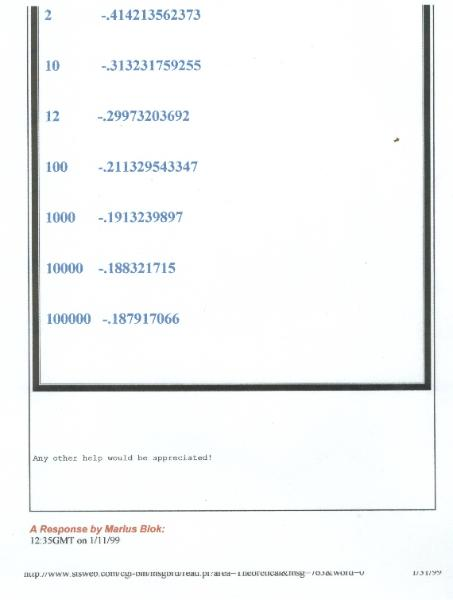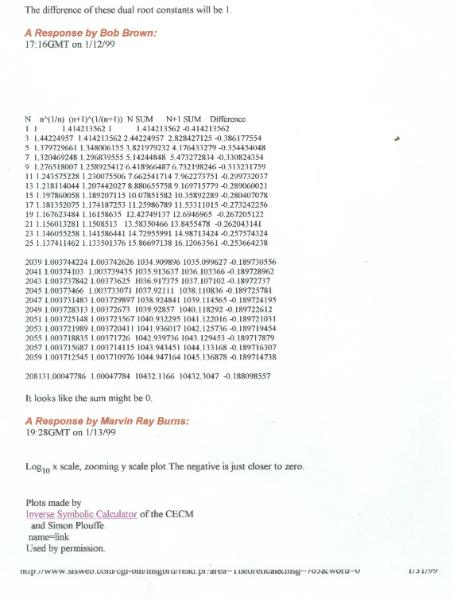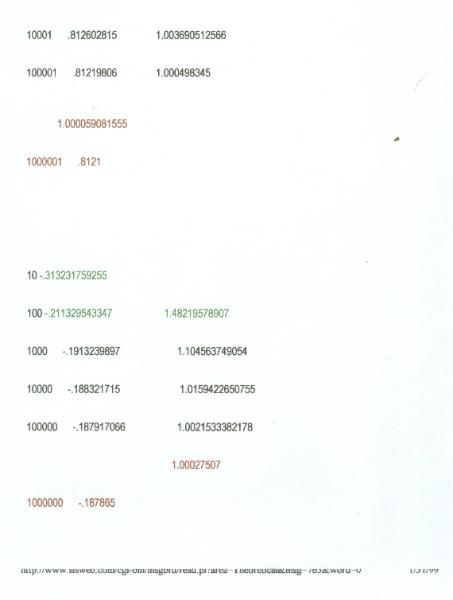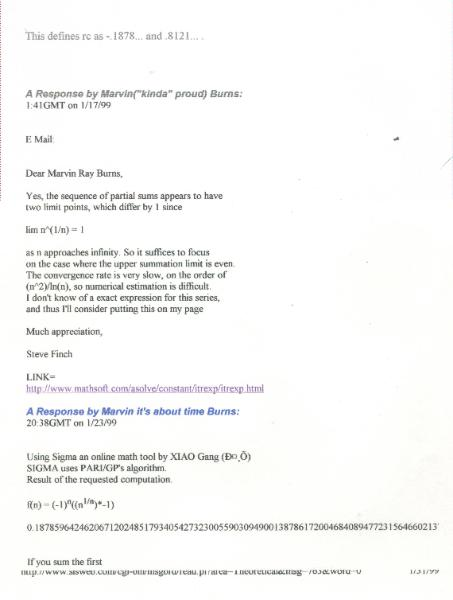The Question by Marvin Ray Burns
3:13GMT on 1/11/99

ROOT CONSTANT
rc

I have started a search for a new
mathematical constant!
Does anyone want to help me?
Consider, 1^(1/1)-2^(1/2)+3^(1/3)...
This may be a contradiction but I call rc
a dual constant.
The root constant for even numbers
(n+1=even number and rc is negative.)
The root constant for odd numbers
(n+1=odd number and rc is positive.)
rc=1^(1/1)-2^(1/2)+3^(1/3)+4^(1/4)...

Table of convergence

Special thanks to
Loki Jorgerson
Centre for Experimental
Constructive Mathematics.
I also used the
Inverse Symbolic Calculator of the
CECM
and Simon Plouffe